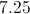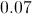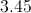# High School Math : Statistical Concepts

## Example Questions

### Example Question #1 : How To Find The Percentiles For A Data Set

The 70th and 80th percentiles of a set of scores are 78 and 86, respectively; the interquartile range of the scores is 41. Which of these scores is more likely than the others to be at the 25th percentile?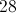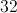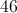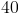Explanation:

The interquartile range of a set of scores is the difference between the third and first quartile - that is, the difference between the 75th and 25th percentiles. The 75th percentile is between 78 and 86, so, if 41 is subtracted from those numbers, the upper and lower bounds of the 25th percentile can be found.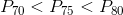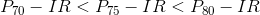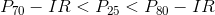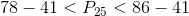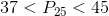Of our choices, only 40 falls in this range.

### Example Question #35 : Basic Statistics

What is the probability of getting a sum of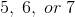when rolling two six-sided fair dice?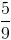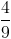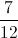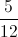Explanation:

The sample space, or total possible outcomes, when rolling two six-sided dice is.

Ways to get what you want: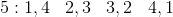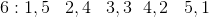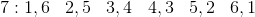So there areways to get a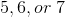.

So the probability becomes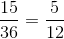### Example Question #36 : Basic Statistics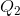is the median of the overall data set.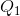is the median of the lower half of the data set.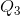is the median of the upper half of the data set.

Find the interquartile range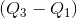for the following data set: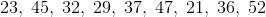Explanation:

First, put the data set in order from smallest to largest: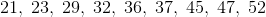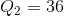which is the middle number in the data set.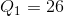which is the average of the two middle numbers of the lower half of the data set.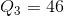which is the average of the two middle numbers of the upper half of the data set.

Thus the interquartile range is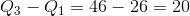Note, the range of the data set is given by the largest number minus the smallest number, or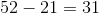### Example Question #37 : Basic Statistics

A bird watcher observed how many birds came to her bird feeder for four days.  These were the results:

Day 1: 15

Day 2: 12

Day 3: 10

Day 4: 13

What is the variance of the number of birds that visited the bird feeder over the four days?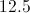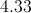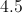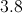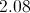Explanation:

Variation measures the average difference between values within a group.  The process is not complicated but there are four steps that can take time.  First, find the mean of the values.  Second, subtract the mean from the first value and square the result.  Do this for all remaining values.  Third, add these results together.  Fourth, divide this value by the number of values in the group minus one (in this case, there are four days).

1: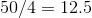2: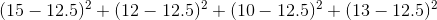3: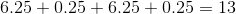4: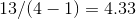Note that to find the standard deviation, we would simply take one additional step of finding the square root of the variance.

### Example Question #38 : Basic Statistics

Alice recorded the outside temperature at noon each day for one week. These were the results.

Monday: 78

Tuesday: 85

Wednesday: 82

Thursday: 84

Friday: 82

Saturday: 79

Sunday: 80

What is the variance of the temperatures?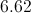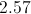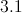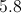Explanation:

There are four steps to finding the variance of values within a group.  First, find the mean of the values.  Second, subtract the mean from the first value and square the result.  Do this for all remaining values.  Third, add these results together.  Fourth, divide the result  by the number of values in the group minus one (in this case, there are seven days, so you must divide by six).

### Example Question #39 : Basic Statistics

The standard deviation of a population is 7.5.  What is the variance of the population?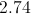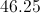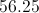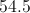Explanation:

This question illustrates the close relationship between the concepts of variance and standard deviation.  We can find variance even though we do not know the values in the population if we know the standard deviation.  Simply square the standard deviation to find the variance.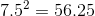### Example Question #1 : Statistical Concepts

A bird watcher observed how many birds came to her bird feeder for four days.  These were the results:

Day 1: 15

Day 2: 12

Day 3: 10

Day 4: 13

Which answer is closest to the standard deviation of the number of birds to visit the bird feeder over the four days?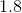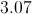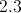Explanation:

Standard deviation is essentially the average distance from the mean of a group of numbers.  There are a number of steps in computing standard deviation, but the steps are not too complicated if you take them one at a time.  First, find the mean of the values.  Second, subtract the mean from the first value and square the result.  Do this for all remaining values.  Third, add these results together.  Fourth, divide this value by the number of values.  Finally, find the square root of the result.

1:2:3:4: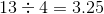5: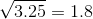### Example Question #2 : Statistical Concepts

Alice recorded the outside temperature at noon each day for one week. These were the results.

Monday: 78

Tuesday: 85

Wednesday: 82

Thursday: 84

Friday: 82

Saturday: 79

Sunday: 80

What is the standard deviation of the temperatures?